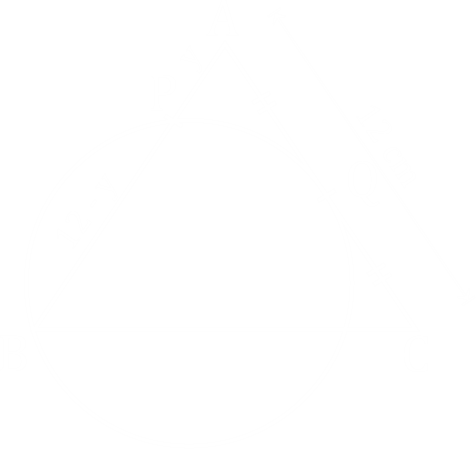Question of The Day30-03-2020

In an isosceles △ ABC, a circle is drawn from the vertex B, which is in between two non-equal sides such that that the circle touches the side opposite to the vertex at its mid-point. The circle also cuts the other equal side at point P. If the equal side of the triangle is 12 cm each, then what will be the value of PB?

Correct Answer : d ) 9

Explanation :

Depicting the given informationIn the above figure we can say that AQC is a tangent to the circle

Thus, applying power of point theorem

(AQ)2 = AP * AB

$$({12 \over 2})^2=y*12$$

⇒ y = 3

Therefore, PB = 12 – y = 12 – 3 = 9

Hence, (d) is the correct answer.

Such type of question is asked in various government exams like SSC CGL, SSC MTS, SSC CPO, SSC CHSL, RRB JE, RRB NTPC, RRB GROUP D, RRB OFFICER SCALE-I, IBPS PO, IBPS SO, RRB Office Assistant, IBPS Clerk, RBI Assistant, IBPS RRB OFFICER SCALE 2&3, UPSC CDS etc.

Read Daily Current Affairs, Banking Awareness, Hindi Current Affairs, Word of the Day, and attempt free mock tests at PendulumEdu and boost your preparation for the actual exam.0